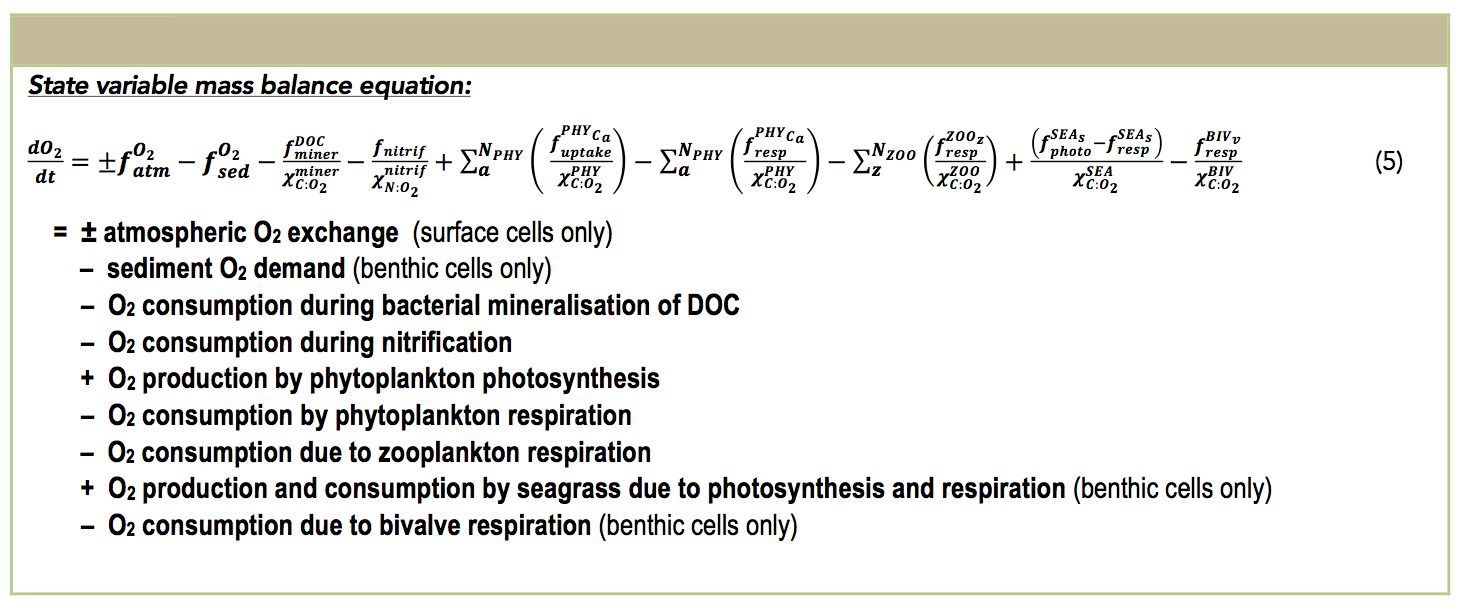﻿ Aquatic Ecodynamics (AED/AED2) model library

## Science Modules / Oxygen

Dissolved Oxygen (DO) dynamics respond to processes of atmospheric exchange, sediment oxygen demand, microbial use during organic matter mineralisation and nitrification, photosynthetic oxygen production and respiratory oxygen consumption, chemical oxygen demand, and respiration by other biotic components such as seagrass and bivalves (see table below).

Other processes impacting the oxygen concentration include the breakdown of DOC by aerobic heterotrophic bacteria to CO2, whereby a stoichiometrically equivalent amount of oxygen is removed. Chemical oxidation, for example processes such as nitrification or sulfide oxidation, also consume oxygen dependent on the relevant stoichiometric factor. Photosynthetic oxygen production and respiratory oxygen consumption by pelagic phytoplankton is also included and is summed over the number of simulated phytoplankton groups. Seagrass interaction with the oxygen cycle is configurable within the model.#### aed2_oxygen : oxygen solubility & atmospheric exchange

Atmospheric exchange is typically modelled based on Wanninkhof (1992) and the flux equation of Riley and Skirrow (1974) for the open water, and on Ho et al. (2011) for estuarine environments.

\begin{equation} F_{O_2} = k_{O_2} \left(C_{air} - C_{water}\right) \end{equation}

where $$k_{O_2} (ms^{-1})$$ is the oxygen transfer coefficient, $$C_{water} (gm^{-3})$$ is the oxygen concentration in the surface waters near the interface and $$C_{air} (gm^{-3})$$ is the concentration of oxygen in the air phase near the interface. A positive flux represents input of oxygen from the atmosphere to the water. $$C_{air}$$ is dependent on temperature, T, salinity, S and atmospheric pressure, p, as given by \cite{Rile74}:

\begin{eqnarray} C_{air}\left(T,S,p \right) &=& 1.42763\: f\left(p\right) \exp \left\{ -173.4292+249.6339 \left[\frac{100}{\theta_k}\right] + 143.3483 \ln \left[ \frac{\theta_k}{100}\right] \right. \nonumber \\ &-& \left. 21.8492 \left[\frac{\theta_k}{100}\right] + S\left(-0.033096 +0.014259 \left[\frac{\theta_k}{100}\right] -0.0017 \left[\frac{\theta_k}{100}\right]^2 \right) \right\} \end{eqnarray} where $$\theta_k$$ is temperature in degrees Kelvin, salinity is expressed as parts per thousand and atmospheric pressure is in kPa. The pressure correction function, f(p) varies between one and zero for the surface and high altitudes respectively: \begin{equation} f\left(p\right)=\frac{p_H}{p_{SL}}\left[1-\frac{p_{vap}}{p_H}\right]/\left[1-\frac{p_{vap}}{p_{SL}}\right]. \end{equation}

#### aed2_oxygen : sediment oxygen demand

Modelling sediment oxygen demand can take a variety of forms. The simplest form in AED2 is the SOD varies as a function of the overlying water temperature and dissolved oxygen levels.

\begin{equation} f_{O_2}^{DSF}(T,DO)= S_{SOD}\:f_{SED}^{T2}(T)\:f_{SOD}^{DO1}(DO) \end{equation} where $$S_{SOD}$$ is a fixed oxygen flux across the sediment-water interface and $$K_{DO_{SOD}}$$ is a half-saturation constant for the sediment oxygen demand.

#### other oxygen sources/sinks (optional)

• Oxygen consumption during baterial mineralisation of DOC is done in the bacteria module - aed2_bacteria;
• Oxygen consumption during nitrification is done in the nitrogen module - aed2_nitrogen;
• Oxygen production/consumption by phytoplankton phtosynthesis/respiration is done in the phytoplankton module - aed2_phytoplankton;
• Oxygen consumption due to zooplankton respiration is done in the zooplankton module - aed2_zooplankton;
• Oxygen production/consumption by seagrass phtosynthesis/respiration is done in the bateria module - aed2_seagrass;
• Oxygen consumption due to bivalve respiration is done in the benthic module - aed2_benthic;

• #### Variable Summary & Setup Options

Variable Name Description Units Variable Type Core/Optional
OXY_oxy dissolved oxygen concentration $$mmol\,m^{-3}$$ pelagic core
Variable Name Description Units Variable Type Core/Optional
OXY_oxysat dissolved oxygen saturation % pelagic diagnostic core
OXY_atm_oxy_flux  O2 exchange across atm/water interface $$mmol\,m^{-2}\,day^{-1}$$ - -
Parameter Name Description Units Parameter Type Default Typical Range Comment
oxy_initial initial dissolved oxygen (DO) concentration $$mmol\,m^{-3}$$ float 0-400 Note: will be overwritten by GLM or TFV IC
Fsed_oxy sediment oxygen demand (SOD) $$mmol\,m^{-2}\,day^{-1}$$ float -100 Note: unused if Fsed_oxy_variable is activated via aed2_sedflux
Ksed_oxy half-saturation concentration of oxygen sediment flux $$mmol\,m^{-3}$$ float 50 10-100 Changes the sensitivity of the oxygen flux to the overlying oxygen concentration
theta_sed_oxy Arrhenius temperature multiplier for sediment oxygen flux - float 1e+00 1.04 - 1.12 Changes the sensitivity of the oxygen flux to the overlying temperature
Fsed_oxy_variable oxygen sediment flux variable link - string ' e.g.: SDF_Fsed_oxy will use the value supplied by the aed2_sedflux model for Fsed_oxy; use this option to allow for spatial or temperal variation
oxy_min minimum dissolved oxygen (DO) concentration $$mmol\,m^{-3}$$ float Optional variable to enforce negative number clipping
oxy_max maxmium dissolved oxygen (DO) concentration $$mmol\,m^{-3}$$ float - 1000 Optional variable to enforce high number clipping

An example nml block for the oxygen module is shown below:

 &aed2_oxygen oxy_initial = 225.0 Fsed_oxy = -40.0 Ksed_oxy = 100.0 theta_sed_oxy = 1.08 ! Fsed_oxy_variable = 'SDF_Fsed_oxy' oxy_min = 0 oxy_max = 500 / 

#### Publications & References

Wanninkhof, R.. 1992. Relationship between windspeed and gas exchange over the ocean. J. Geophys. Res. (Oceans) 97(C5): 7373– 7382.

Riley, J.P. & Skirrow, G.. 1974. Chemical Oceanography Academic Press, London.

Ho, D.T., Schlosser P., & Orton, P.M., 2011. On factors controlling air-water gas exchange in a large tidal river. Estuaries and Coasts. 34:1103-1116.